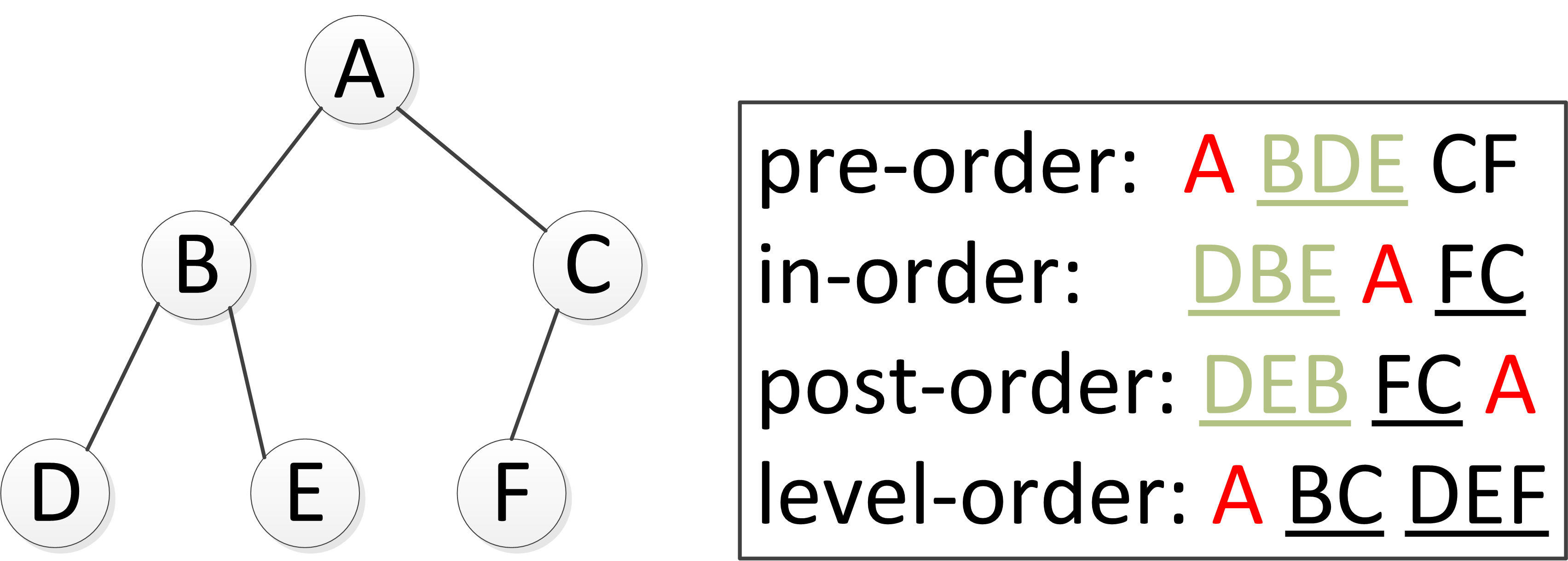# Binary Tree - 二元樹

## 程式實現

### Python

class TreeNode:
def __init__(self, val):
self.val = val
self.left, self.right = None, None


### C++

struct TreeNode {
int val;
TreeNode *left;
TreeNode *right;
TreeNode(int x) : val(x), left(NULL), right(NULL) {}
};


### Java

public class TreeNode {
public int val;
public TreeNode left, right;
public TreeNode(int val) {
this.val = val;
this.left = null;
this.right = null;
}
}


## Tree traversal 樹的遍歷

• 深度優先(depth-first)：先訪問子節點，再訪問父節點，最後訪問第二個子節點。根據根節點相對於左右子節點的訪問先後順序又可細分為以下三種方式。
1. 前序(pre-order)：先根後左再右
2. 中序(in-order)：先左後根再右
3. 後序(post-order)：先左後右再根### Python

class TreeNode:
def __init__(self, val):
self.val = val
self.left, self.right = None, None

class Traversal(object):
def __init__(self):
self.traverse_path = list()

def preorder(self, root):
if root:
self.traverse_path.append(root.val)
self.preorder(root.left)
self.preorder(root.right)

def inorder(self,root):
if root:
self.inorder(root.left)
self.traverse_path.append(root.val)
self.inorder(root.right)

def postorder(self,root):
if root:
self.postorder(root.left)
self.postorder(root.right)
self.traverse_path.append(root.val)